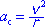a-sub-c = r times omega-squared

centripetal acceleration equals radius times angular-velocity-squared

A point particle that is going around in a circle has an acceleration that is directed toward the center of the circle and that has a magnitude equal to the product of the radius of the circle and the square of the magnitude of the angular velocity of an imaginary line extending from the center of the circle to the particle. Centripetal acceleration can also be expressed as:(1)

Note: The expression at the top of this page is easily derived from equation (1) by substitutingfor v in equation (1).

Note: We generally think of the angular velocity, omega, as characterizing an object that is spinning (rotating) on an axis rather than a point particle that is going around in a circle. In the case of a point particle going around in a circle, an imaginary line extending from the axis of rotation to the point particle is indeed rotating (in a manner similar to the way the second hand on a clock rotates). Thus we can use angular velocity (spin rate) to characterize the particle going around in a circle. Typically we do call such an angular velocity the angular velocity of the particle but it means the same thing as the angular velocity of the imaginary line that extends from the center of the circle to the particle.